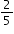# MAFS.912.G-GPE.2.6Archived StandardExport Print
Find the point on a directed line segment between two given points that partitions the segment in a given ratio.
General Information
Subject Area: Mathematics
Domain-Subdomain: Geometry: Expressing Geometric Properties with Equations
Cluster: Level 1: Recall
Cluster: Use coordinates to prove simple geometric theorems algebraically. (Geometry - Major Cluster) -

Clusters should not be sorted from Major to Supporting and then taught in that order. To do so would strip the coherence of the mathematical ideas and miss the opportunity to enhance the major work of the grade with the supporting clusters.

Date of Last Rating: 02/14
Status: State Board Approved - Archived
Assessed: Yes
Test Item Specifications

• Assessment Limits :

None

• Calculator :

Neutral

• Clarification :
Students will find a point on a directed line segment between two
given points when given the partition as a ratio.
• Stimulus Attributes :
Items may be set in a real-world or mathematical context.
• Response Attributes :
Items may require the student to find a ratio when given the
endpoints of a directed line segment and a point on the line segment.

Items may require the student to find an endpoint when given a ratio,
one endpoint, and a point on the directed line segment.

Sample Test Items (1)
• Test Item #: Sample Item 1
• Question:

Points A,B, and C are colinear and AB:BC =. Point A is located at (-3,6), point B is located at (n,q), and point C is located at (-3,-4).

What are the values of n and q?

• Difficulty: N/A
• Type: EE: Equation Editor

## Related Courses

This benchmark is part of these courses.
1200400: Foundational Skills in Mathematics 9-12 (Specifically in versions: 2014 - 2015, 2015 - 2022, 2022 and beyond (current))
1206300: Informal Geometry (Specifically in versions: 2014 - 2015, 2015 - 2022 (course terminated))
1206310: Geometry (Specifically in versions: 2014 - 2015, 2015 - 2022, 2022 and beyond (current))
1206320: Geometry Honors (Specifically in versions: 2014 - 2015, 2015 - 2022, 2022 and beyond (current))
1200700: Mathematics for College Algebra (Specifically in versions: 2014 - 2015, 2015 - 2022 (course terminated))
7912060: Access Informal Geometry (Specifically in versions: 2014 - 2015 (course terminated))
1206315: Geometry for Credit Recovery (Specifically in versions: 2014 - 2015, 2015 - 2022, 2022 and beyond (current))
7912065: Access Geometry (Specifically in versions: 2015 - 2022, 2022 and beyond (current))

## Related Access Points

Alternate version of this benchmark for students with significant cognitive disabilities.

## Related Resources

Vetted resources educators can use to teach the concepts and skills in this benchmark.

## Formative Assessments

Partitioning a Segment:

Students are asked to find the coordinates of a point which partitions a segment in a given ratio.

Type: Formative Assessment

Centroid Coordinates:

Students are asked to find the coordinates of the centroid when given the ratio of a directed segment.

Type: Formative Assessment

## Lesson Plans

Keeping Triangles in Balance: Discovering Triangle Centroid is Concurrent Medians:

In this lesson, students identify, analyze, and understand the Triangle Centroid Theorem. Students discover that the centroid is the point of concurrency for the medians of a triangle and recognize its associated usage with the center of gravity or barycenter. This set of instructional materials provides the teacher with hands-on activities using technology as well as paper-and-pencil methods.

Type: Lesson Plan

Partition Point For The Queen:

Students will use a variety of methods to locate a point to partition a line segment to a given ratio. The activities will range from informal student definitions and sketches to tasks using number lines and the coordinate plane.

Type: Lesson Plan

Geometree Thievery:

This geometry lesson focuses on partitioning a segment on a coordinate grid in a non-traditional and interesting format. Students will complete a series of problems to determine which farmers are telling the truth about their harvested "Geometrees."

Type: Lesson Plan

Partitioning a Segment:

In this lesson, students find the point on a directed line segment between two given points that partitions the segment in a given ratio.

Type: Lesson Plan

Partition Me:

Partition, what's that? Well come find out as you learn to break it down, a segment that is. Learn the basic skills of partitioning and you can turn your class into a partitioning party; just BYOGP (Bring your own graph paper).

Type: Lesson Plan

## Original Student Tutorial

High Tech Seesaw:

Learn how to find the point on a directed line segment that partitions it into a given ratio in this interactive tutorial.

Type: Original Student Tutorial

## Perspectives Video: Professional/Enthusiast

Amping Up Violin Tuning with Math:

Kyle Dunn, a Tallahassee-based luthier and owner of Stringfest, discusses how math is related to music.

Type: Perspectives Video: Professional/Enthusiast

## MFAS Formative Assessments

Centroid Coordinates:

Students are asked to find the coordinates of the centroid when given the ratio of a directed segment.

Partitioning a Segment:

Students are asked to find the coordinates of a point which partitions a segment in a given ratio.

## Original Student Tutorials Mathematics - Grades 9-12

High Tech Seesaw:

Learn how to find the point on a directed line segment that partitions it into a given ratio in this interactive tutorial.

## Student Resources

Vetted resources students can use to learn the concepts and skills in this benchmark.

## Original Student Tutorial

High Tech Seesaw:

Learn how to find the point on a directed line segment that partitions it into a given ratio in this interactive tutorial.

Type: Original Student Tutorial

## Parent Resources

Vetted resources caregivers can use to help students learn the concepts and skills in this benchmark.